##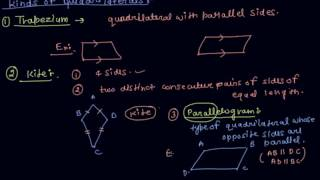By Scholarslearning Classes

Properties of Quadrilaterals | Class 8 Mathematics Understanding Quadrilaterals by Pratyanshu Porwal##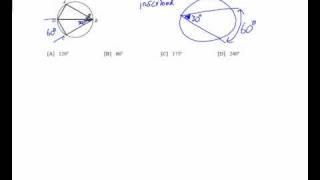By mathrageous

Accelerated Math Video for objective "Inscribed Quadrilaterals"# 5.7 Proving that Figures are Special Quadrilaterals (Practice)

##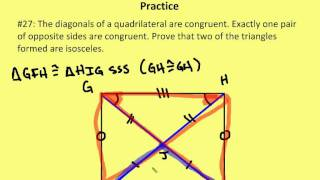By AutenMath

5.7 Proving that Figures are Special Quadrilaterals (Practice)##By Tim Bedley

Tim Bedley veteran teacher gives a mini lesson to remind students how to recognize a quadrilateral. This is a great resource to introduce or to review quadrilaterals in the elementary classroom.##By google infinite

A polygon is any 2-dimensional shape formed with straight lines. Triangles, quadrilaterals, pentagons, and hexagons are all examples of polygons.# NCERT- Class 8 Maths -Understanding Quadrilaterals – Exe.1 -Que. 7

##By LakshyaEducation##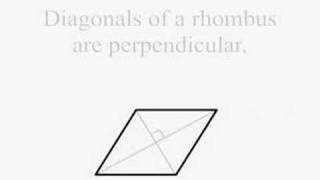By Matthew Marden

Video that plays music and goes through slides describing the properties of a quadrilateral.##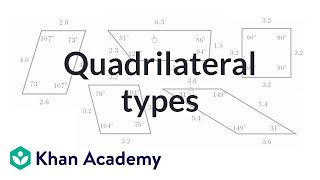By Khan Academy

Take a look at the given data and determine what type of quadrilateral we have.##By Khan Academy

"Quad" means "four" and "lateral" means "line." A quadrilateral is literally a shape with four sides (lines) and four angles. Let's learn the difference between concave and convex quadrilaterals as well as trapezoids parallelograms and rhombi.##By Khan Academy##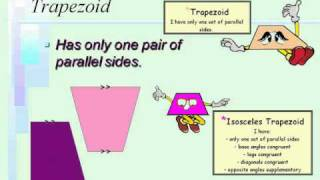By MathLambert

Video slides and explanations on classifying quadrilaterals.# Sum of all angles in quadrilateral is 360° (Theorem and Proof)

##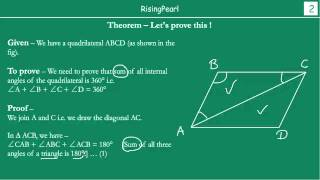By dostotussigreatho

This is a Math video. This is our third webisode (WB-3) on "Series 8 -- Quadrilaterals". In this video, we learn that sum of all four internal angles in a quadrilateral is 360°. Then we go ahead and prove this theorem.##By EducationCommonsRW

This lesson reviews and describes the properties of all types of quadrilaterals. It also demonstrates how to compare the properties of different quadrilaterals with each other.# 5.7 Proving that Figures are Special Quadrilaterals (Lesson)

##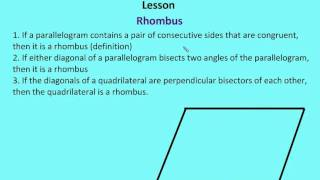By AutenMath

5.7 Proving that Figures are Special Quadrilaterals (Lesson)##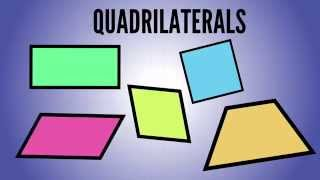By Dylan Peters EDU

Students will be able to identify and classify quadrilaterals based on the sides, angles, and other properties.##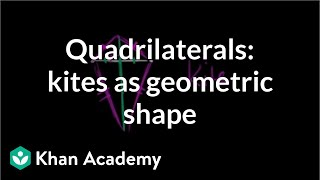By Khan Academy

Is a kite a quadrilateral? Is it a rhombus? A parallelogram? Let's find out.# Quadrilateral problems on the coordinate plane

##By Khan Academy

Sal solves a challenging problem involving a quadrilateral on the coordinate plane.# Understanding Quadrilaterals | Class 8th Mathematics | NCERT | CBSE Syllabus | Live Videos

##By Pebbles CBSE Board Syllabus

Understanding Quadrilaterals | Class 8th Mathematics | NCERT | CBSE Syllabus | Live Videos This video is a part of Pebbles CBSE Syllabus Live Teaching Videos Pack.# Maths Practical Geometry part 2 (Quadrilateral with 4 sides & 1 diagonal) CBSE Class 8 Mathematics

##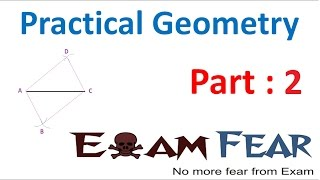By ExamFear Education

Maths Practical Geometry part 2 (Quadrilateral with 4 sides & 1 diagonal) CBSE Class 8 Mathematics# Quadrilateral properties | Perimeter, area, and volume | Geometry | Khan Academy

##By Khan Academy